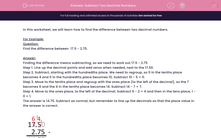# Subtract Two Decimal Numbers

In this worksheet, students practise subtracting decimal numbers.Key stage:  KS 2

Curriculum topic:   Maths and Numerical Reasoning

Curriculum subtopic:   Decimals

Difficulty level:#### Worksheet Overview

In this worksheet, we will learn how to find the difference between two decimal numbers.

For Example:

Question:

Find the difference between  17.5 - 2.75.

Finding the difference means subtracting, so we need to work out 17.5 - 2.75

Step 1. Line up the decimal points and add zeros when needed, next to the 17.50.

Step 2. Subtract, starting with the hundredths place. We need to regroup, so 5 in the tenths place becomes 4 and 0 in the hundredths place becomes 10. Subtract 10 - 5 = 5.

Step 3. Move to the tenths place and regroup with the ones place (to the left of the decimal), so the 7 becomes 6 and the 4 in the tenths place becomes 14. Subtract 14 - 7 = 7.

Step 4. Move to the ones place, to the left of the decimal. Subtract 6 - 2 = 4 and then in the tens place, 1 - 0 = 1.

The answer is 14.75. Subtract as normal, but remember to line up the decimals so that the place value in the answer is correct.### What is EdPlace?

We're your National Curriculum aligned online education content provider helping each child succeed in English, maths and science from year 1 to GCSE. With an EdPlace account you’ll be able to track and measure progress, helping each child achieve their best. We build confidence and attainment by personalising each child’s learning at a level that suits them.

Get startedTry an activity or get started for free